# 一看就懂的感知机算法PLA机器学习 同时被 3 个专栏收录74 篇文章 9 订阅

CSDN博客：红色石头的专栏

GitHub：RedstoneWill的GitHub

## 什么是感知机「Perceptron」

PLA全称是Perceptron Linear Algorithm，即线性感知机算法，属于一种最简单的感知机（Perceptron）模型。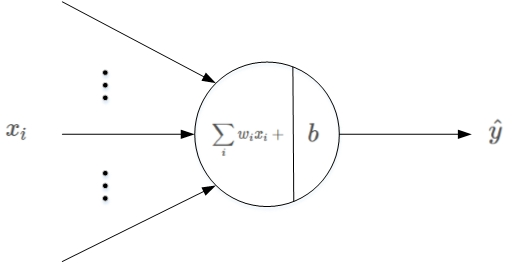$scores=\sum _{i}^{N}{w}_{i}{x}_{i}+b$

$scores=\sum _{i}^{N+1}{w}_{i}{x}_{i}$

scores s c o r e s $scores$是感知机的输出，接下来就要对 scores s c o r e s $scores$进行判断：

• scores0 s c o r e s ≥ 0 $scores\geq0$，则 y^=1 y ^ = 1 $\hat y=1$（正类）

• scores<0 s c o r e s < 0 $scores<0$，则 y^=1 y ^ = − 1 $\hat y=-1$（负类）

## PLA理论解释

w:=w+x=w+yx w := w + x = w + y x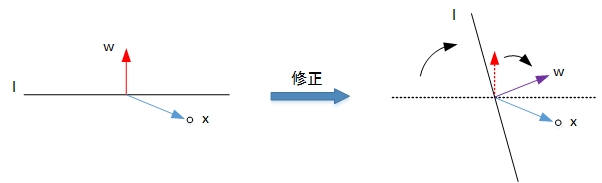w:=wx=w+yx w := w − x = w + y x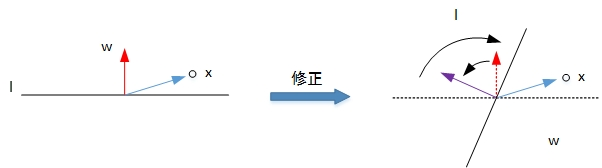## 数据准备

### 导入数据

import numpy as np
import pandas as pd

# 样本输入，维度（100，2）
X = data.iloc[:,:2].values
# 样本输出，维度（100，）
y = data.iloc[:,2].values

### 数据分类与可视化

import matplotlib.pyplot as plt

plt.scatter(X[:50, 0], X[:50, 1], color='blue', marker='o', label='Positive')
plt.scatter(X[50:, 0], X[50:, 1], color='red', marker='x', label='Negative')
plt.xlabel('Feature 1')
plt.ylabel('Feature 2')
plt.legend(loc = 'upper left')
plt.title('Original Data')
plt.show()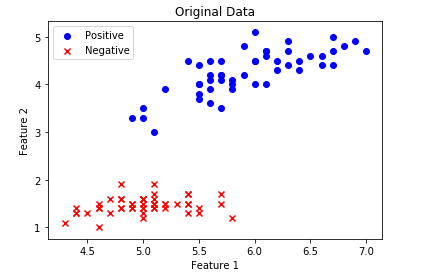## PLA算法

### 特征归一化

$X=\frac{X-\mu }{\sigma }$

# 均值
u = np.mean(X, axis=0)
# 方差
v = np.std(X, axis=0)

X = (X - u) / v

# 作图
plt.scatter(X[:50, 0], X[:50, 1], color='blue', marker='o', label='Positive')
plt.scatter(X[50:, 0], X[50:, 1], color='red', marker='x', label='Negative')
plt.xlabel('Feature 1')
plt.ylabel('Feature 2')
plt.legend(loc = 'upper left')
plt.title('Normalization data')
plt.show()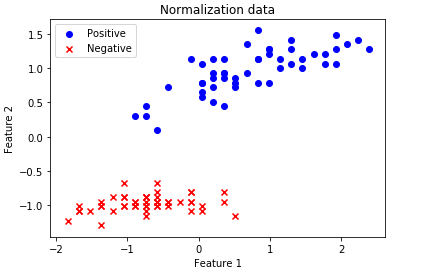### 直线初始化

# X加上偏置项
X = np.hstack((np.ones((X.shape,1)), X))
# 权重初始化
w = np.random.randn(3,1)

# 直线第一个坐标（x1，y1）
x1 = -2
y1 = -1 / w * (w * 1 + w * x1)
# 直线第二个坐标（x2，y2）
x2 = 2
y2 = -1 / w * (w * 1 + w * x2)
# 作图
plt.scatter(X[:50, 1], X[:50, 2], color='blue', marker='o', label='Positive')
plt.scatter(X[50:, 1], X[50:, 2], color='red', marker='x', label='Negative')
plt.plot([x1,x2], [y1,y2],'r')
plt.xlabel('Feature 1')
plt.ylabel('Feature 2')
plt.legend(loc = 'upper left')
plt.show()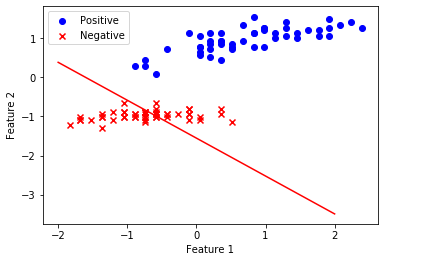### 计算scores，更新权重

s = np.dot(X, w)
y_pred = np.ones_like(y)    # 预测输出初始化
loc_n = np.where(s < 0)    # 大于零索引下标
y_pred[loc_n] = -1

# 第一个分类错误的点
t = np.where(y != y_pred)
# 更新权重w
w += y[t] * X[t, :].reshape((3,1))

### 迭代更新训练

for i in range(100):
s = np.dot(X, w)
y_pred = np.ones_like(y)
loc_n = np.where(s < 0)
y_pred[loc_n] = -1
num_fault = len(np.where(y != y_pred))
print('第%2d次更新，分类错误的点个数：%2d' % (i, num_fault))
if num_fault == 0:
break
else:
t = np.where(y != y_pred)
w += y[t] * X[t, :].reshape((3,1))

# 直线第一个坐标（x1，y1）
x1 = -2
y1 = -1 / w * (w * 1 + w * x1)
# 直线第二个坐标（x2，y2）
x2 = 2
y2 = -1 / w * (w * 1 + w * x2)
# 作图
plt.scatter(X[:50, 1], X[:50, 2], color='blue', marker='o', label='Positive')
plt.scatter(X[50:, 1], X[50:, 2], color='red', marker='x', label='Negative')
plt.plot([x1,x2], [y1,y2],'r')
plt.xlabel('Feature 1')
plt.ylabel('Feature 2')
plt.legend(loc = 'upper left')
plt.show()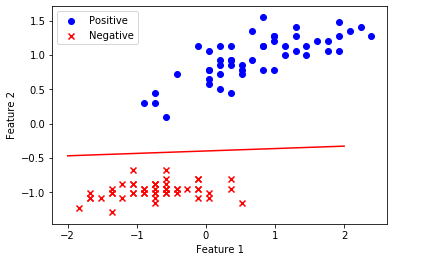## 总结与疑问09-20
01-111万+12-30644
03-151万+
11-241422
12-232130
10-261055
10-101213
07-043219
04-163811
04-10621
03-14733
11-276894
09-293万+
07-28
05-308083
08-061325
11-21
04-232万+点击重新获取扫码支付余额充值